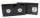# Mixing Celsius and Fahrenheit

Add up three temperatures:

5°F +6°F +0°C

Result

t1 =  43 °F
t2 =  -11.667 °C

#### Solution:Leave us a comment of example and its solution (i.e. if it is still somewhat unclear...):

Showing 1 comment:Math student
Hi
The above solution is incorrect as 0 degree Celsius is 32 degree Fahrenheit. Hence, the answer is 5 degree F + 6 degree F + 32 degree F = 43 degree F

Thank you## Next similar examples:

1. If the 2If the temperature is 86 degrees in Fahrenheit, what is the same temperature in Celsius?
2. 8 degrees8 degrees Celsius it cools down by 9 degrees Celsius. What is the resultant temperature?
3. Temperature conversionThe normal human body temperature is 98.6 degrees Fahrenheit. What is the temperature in degrees Celcius?
4. Adding mixed numerals3 3/4 + 2 3/5 + 5 1/2 Show your solution.
5. On SundayOn Sunday the temperature reached a high of 38° above 0. That night it dropped to 23° below 0. What is the difference between the high and low temperatures for Sunday?
6. The lowestThe lowest temperature on Thursday was 20°C. The lowest temperature on Saturday was 12°C. What was the difference between the lowest temperatures?
7. VideotapeViera bought a videotape on which you can record programs with a total length of 240 minutes. She recorded a sci fi movie 1 hour and 28 minutes long, five ten-minute sessions "aerobics at home." Can she fits on the tape even film of Robin Hood who takes a
8. Pound2kilosHow many pounds make 1 kilograms?
9. Customary lengthConvert length 65yd 2 ft to ft
10. RapeThe agricultural cooperative harvested 525 ares of rape, of which received 5.6 tons of rape seeds. Calculate the yield per hectare of rape.
11. NoontimeIn the morning temperature was 110°F. By noontime it has gone up by 15°F. What was the noon temperature. (Give your answer in °F)
12. OpheliaOphelia recorded the temperature of a cold store every two hours. 1. At 6am it was -4°C and at 8am it was -1°C. By how much did the temperature rise? 2. The temperature went up by 5°C in the next two hours. .What was the temperature at 10am?
13. LaboratoryMang Elio went to his laboratory at 7:00am And recorded that the temperature was 11.07°C. At lunchtime, the temperature rose by 27.9°C but dropped by 8.05°C at 5:00 PM. What was the temperature at 5:00 PM?
14. This morningThis morning it was 80 degrees F outside the temperature increased 12 degrees F. What is the temperature now?
15. Combine / add termCombine like terms 4c+c-7c
16. Sum of fractionsWhat is the sum of 2/3+3/5?
17. Evaluate expression 2Evaluate expression with negatives: (-3)+4+(-8)+(-6)+4+(-1)